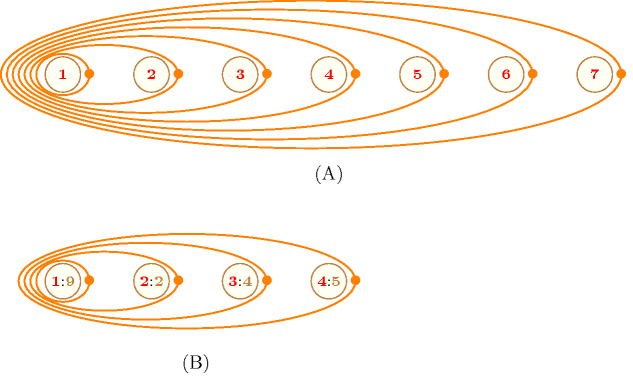## 5.348. size_max_starting_seq_alldifferent

Origin
Constraint

$\mathrm{𝚜𝚒𝚣𝚎}_\mathrm{𝚖𝚊𝚡}_\mathrm{𝚜𝚝𝚊𝚛𝚝𝚒𝚗𝚐}_\mathrm{𝚜𝚎𝚚}_\mathrm{𝚊𝚕𝚕𝚍𝚒𝚏𝚏𝚎𝚛𝚎𝚗𝚝}\left(\mathrm{𝚂𝙸𝚉𝙴},\mathrm{𝚅𝙰𝚁𝙸𝙰𝙱𝙻𝙴𝚂}\right)$

Synonyms

$\mathrm{𝚜𝚒𝚣𝚎}_\mathrm{𝚖𝚊𝚡𝚒𝚖𝚊𝚕}_\mathrm{𝚜𝚝𝚊𝚛𝚝𝚒𝚗𝚐}_\mathrm{𝚜𝚎𝚚𝚞𝚎𝚗𝚌𝚎}_\mathrm{𝚊𝚕𝚕𝚍𝚒𝚏𝚏}$, $\mathrm{𝚜𝚒𝚣𝚎}_\mathrm{𝚖𝚊𝚡𝚒𝚖𝚊𝚕}_\mathrm{𝚜𝚝𝚊𝚛𝚝𝚒𝚗𝚐}_\mathrm{𝚜𝚎𝚚𝚞𝚎𝚗𝚌𝚎}_\mathrm{𝚊𝚕𝚕𝚍𝚒𝚜𝚝𝚒𝚗𝚌𝚝}$, $\mathrm{𝚜𝚒𝚣𝚎}_\mathrm{𝚖𝚊𝚡𝚒𝚖𝚊𝚕}_\mathrm{𝚜𝚝𝚊𝚛𝚝𝚒𝚗𝚐}_\mathrm{𝚜𝚎𝚚𝚞𝚎𝚗𝚌𝚎}_\mathrm{𝚊𝚕𝚕𝚍𝚒𝚏𝚏𝚎𝚛𝚎𝚗𝚝}$.

Arguments
 $\mathrm{𝚂𝙸𝚉𝙴}$ $\mathrm{𝚍𝚟𝚊𝚛}$ $\mathrm{𝚅𝙰𝚁𝙸𝙰𝙱𝙻𝙴𝚂}$ $\mathrm{𝚌𝚘𝚕𝚕𝚎𝚌𝚝𝚒𝚘𝚗}\left(\mathrm{𝚟𝚊𝚛}-\mathrm{𝚍𝚟𝚊𝚛}\right)$
Restrictions
 $\mathrm{𝚂𝙸𝚉𝙴}\ge 0$ $\mathrm{𝚂𝙸𝚉𝙴}\le |\mathrm{𝚅𝙰𝚁𝙸𝙰𝙱𝙻𝙴𝚂}|$ $\mathrm{𝚛𝚎𝚚𝚞𝚒𝚛𝚎𝚍}$$\left(\mathrm{𝚅𝙰𝚁𝙸𝙰𝙱𝙻𝙴𝚂},\mathrm{𝚟𝚊𝚛}\right)$
Purpose

$\mathrm{𝚂𝙸𝚉𝙴}$ is the size of the maximal sequence (among all sequences of consecutive variables of the collection $\mathrm{𝚅𝙰𝚁𝙸𝙰𝙱𝙻𝙴𝚂}$ starting at position one) for which the $\mathrm{𝚊𝚕𝚕𝚍𝚒𝚏𝚏𝚎𝚛𝚎𝚗𝚝}$ constraint holds.

Example
 $\left(4,〈9,2,4,5,2,7,4〉\right)$ $\left(7,〈9,2,4,5,1,7,8〉\right)$ $\left(6,〈9,2,4,5,1,7,9〉\right)$

The first $\mathrm{𝚜𝚒𝚣𝚎}_\mathrm{𝚖𝚊𝚡}_\mathrm{𝚜𝚝𝚊𝚛𝚝𝚒𝚗𝚐}_\mathrm{𝚜𝚎𝚚}_\mathrm{𝚊𝚕𝚕𝚍𝚒𝚏𝚏𝚎𝚛𝚎𝚗𝚝}$ constraint holds since the constraint $\mathrm{𝚊𝚕𝚕𝚍𝚒𝚏𝚏𝚎𝚛𝚎𝚗𝚝}$$\left(〈\mathrm{𝚟𝚊𝚛}-9,\mathrm{𝚟𝚊𝚛}-2,\mathrm{𝚟𝚊𝚛}-4,\mathrm{𝚟𝚊𝚛}-5〉\right)$ holds and since $\mathrm{𝚊𝚕𝚕𝚍𝚒𝚏𝚏𝚎𝚛𝚎𝚗𝚝}$$\left(〈\mathrm{𝚟𝚊𝚛}-9,\mathrm{𝚟𝚊𝚛}-2,\mathrm{𝚟𝚊𝚛}-4,\mathrm{𝚟𝚊𝚛}-5,\mathrm{𝚟𝚊𝚛}-2〉\right)$ does not hold.

Typical
 $\mathrm{𝚂𝙸𝚉𝙴}>2$ $\mathrm{𝚂𝙸𝚉𝙴}<|\mathrm{𝚅𝙰𝚁𝙸𝙰𝙱𝙻𝙴𝚂}|$ $\mathrm{𝚛𝚊𝚗𝚐𝚎}$$\left(\mathrm{𝚅𝙰𝚁𝙸𝙰𝙱𝙻𝙴𝚂}.\mathrm{𝚟𝚊𝚛}\right)>1$
Symmetry

One and the same constant can be added to the $\mathrm{𝚟𝚊𝚛}$ attribute of all items of $\mathrm{𝚅𝙰𝚁𝙸𝙰𝙱𝙻𝙴𝚂}$.

Arg. properties

Functional dependency: $\mathrm{𝚂𝙸𝚉𝙴}$ determined by $\mathrm{𝚅𝙰𝚁𝙸𝙰𝙱𝙻𝙴𝚂}$.

Remark

A conditional constraint [MittalFalkenhainer90] with the specific structure that one can relax the constraints on the last variables of the collection $\mathrm{𝚅𝙰𝚁𝙸𝙰𝙱𝙻𝙴𝚂}$.

Counting
 Length ($n$) 2 3 4 5 6 7 8 Solutions 9 64 625 7776 117649 2097152 43046721

Number of solutions for $\mathrm{𝚜𝚒𝚣𝚎}_\mathrm{𝚖𝚊𝚡}_\mathrm{𝚜𝚝𝚊𝚛𝚝𝚒𝚗𝚐}_\mathrm{𝚜𝚎𝚚}_\mathrm{𝚊𝚕𝚕𝚍𝚒𝚏𝚏𝚎𝚛𝚎𝚗𝚝}$: domains $0..n$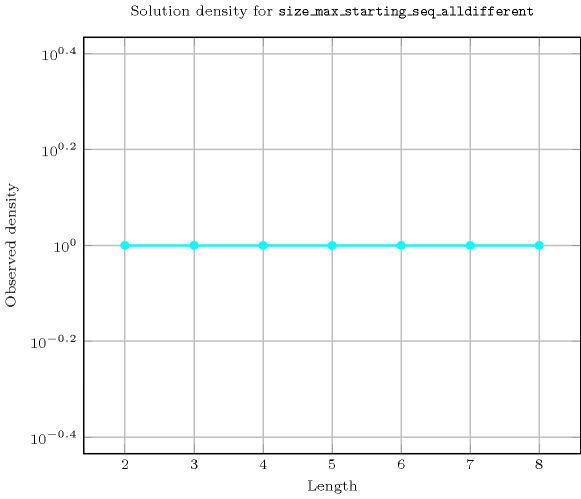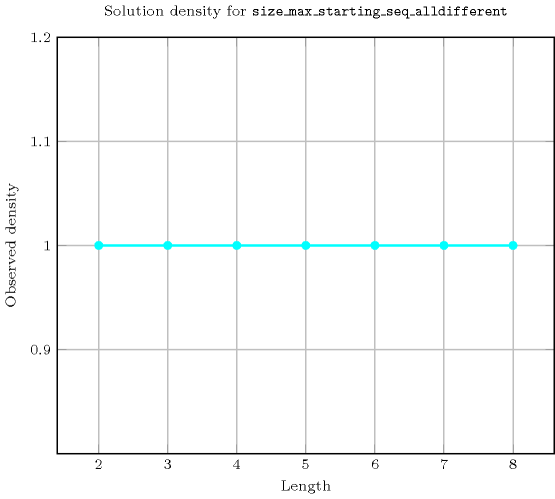Length ($n$)2345678
Total9646257776117649209715243046721
 Parameter value

13161251296168072621444782969
26242002160288124587528503056
3-241802160308705160969920232
4--1201440235204300808817984
5---720126002688006123600
6----50401209603265920
7-----403201270080
8------362880

Solution count for $\mathrm{𝚜𝚒𝚣𝚎}_\mathrm{𝚖𝚊𝚡}_\mathrm{𝚜𝚝𝚊𝚛𝚝𝚒𝚗𝚐}_\mathrm{𝚜𝚎𝚚}_\mathrm{𝚊𝚕𝚕𝚍𝚒𝚏𝚏𝚎𝚛𝚎𝚗𝚝}$: domains $0..n$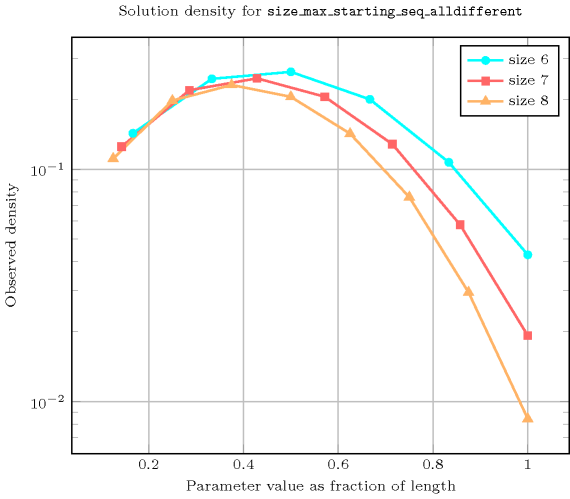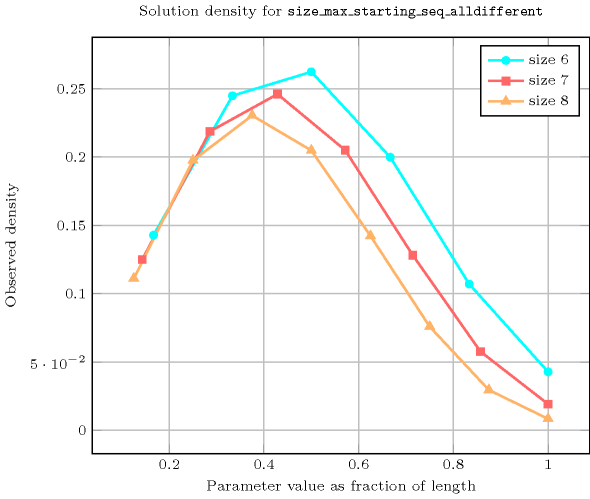Keywords
Arc input(s)

$\mathrm{𝚅𝙰𝚁𝙸𝙰𝙱𝙻𝙴𝚂}$

Arc generator
$\mathrm{𝑃𝐴𝑇𝐻}_\mathit{1}$$↦\mathrm{𝚌𝚘𝚕𝚕𝚎𝚌𝚝𝚒𝚘𝚗}$

Arc arity
$*$
Arc constraint(s)
$\mathrm{𝚊𝚕𝚕𝚍𝚒𝚏𝚏𝚎𝚛𝚎𝚗𝚝}$$\left(\mathrm{𝚌𝚘𝚕𝚕𝚎𝚌𝚝𝚒𝚘𝚗}\right)$
Graph property(ies)
$\mathrm{𝐍𝐀𝐑𝐂}$$=\mathrm{𝚂𝙸𝚉𝙴}$

Graph model

Note that this is an example where the arc constraints do not have the same arity. However they correspond to the same constraint.

Parts (A) and (B) of Figure 5.348.1 respectively show the initial and final graph associated with the first example of the Example slot.

##### Figure 5.348.1. (A) Initial and (B) final graph of the $\mathrm{𝚜𝚒𝚣𝚎}_\mathrm{𝚖𝚊𝚡}_\mathrm{𝚜𝚝𝚊𝚛𝚝𝚒𝚗𝚐}_\mathrm{𝚜𝚎𝚚}_\mathrm{𝚊𝚕𝚕𝚍𝚒𝚏𝚏𝚎𝚛𝚎𝚗𝚝}$$\left(\mathbf{4},〈9,2,4,5,2,7,4〉\right)$ constraint of the first example of the Example slot where each ellipse represents an hyperedge corresponding to an $\mathrm{𝚊𝚕𝚕𝚍𝚒𝚏𝚏𝚎𝚛𝚎𝚗𝚝}$ constraint (e.g., the fourth ellipse represents the constraint $\mathrm{𝚊𝚕𝚕𝚍𝚒𝚏𝚏𝚎𝚛𝚎𝚗𝚝}$$〈9,2,4,5〉$)